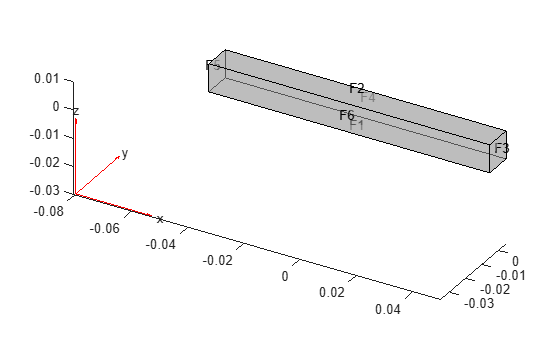# ReducedStructuralModel

Reduced-order structural model results

Since R2019b

## Description

A `ReducedStructuralModel` object contains the stiffness matrix K, mass matrix M, mesh, multipoint constraint reference locations, and IDs of retained degrees of freedom.

To expand this data to a full solution that includes displacement, velocity, and acceleration, use `reconstructSolution`.

## Creation

Reduce a structural model by using the `reduce` function. This function returns structural results obtained using the Craig-Bampton reduced order method as a `ReducedStructuralModel` object.

## Properties

expand all

Reduced stiffness matrix, specified as a real `N`-by-`N` matrix.

• For models without multipoint constraints, `N` is the sum of the number of retained degrees of freedom and the number of fixed interface modes.

• For models with `Nmp` multipoint constraints, `N` is the sum of `6*Nmp` and the number of fixed interface modes.

Data Types: `double`

Reduced mass matrix, specified as a real `N`-by-`N` matrix.

• For models without multipoint constraints, `N` is the sum of the number of retained degrees of freedom and the number of fixed interface modes.

• For models with `Nmp` multipoint constraints, `N` is the sum of `6*Nmp` and the number of fixed interface modes.

Data Types: `double`

Number of fixed interface modes, specified as an integer.

Data Types: `double`

IDs of retained degrees of freedom, specified as a real vector.

Data Types: `double`

Multipoint constraint reference locations, specified as a real `2`-by-`Nmp` or `3`-by-`Nmp` matrix for a 2-D or 3-D geometry, respectively. Here, `Nmp` is the number of multipoint constraints. If there are no multipoint constraints, `ReferenceLocations` is an empty matrix.

Data Types: `double`

Finite element mesh, specified as a `FEMesh` object. For details, see FEMesh Properties.

## Object Functions

 `reconstructSolution` Recover full-model transient solution from reduced-order model (ROM)

## Examples

collapse all

Reduce a transient structural model to the fixed interface modes in a specified frequency range and the boundary interface degrees of freedom.

Create a transient structural model for a 3-D problem.

`structuralmodel = createpde("structural","transient-solid");`

Create a geometry and include it in the model. Plot the geometry.

```gm = multicuboid(0.1,0.01,0.01); structuralmodel.Geometry = gm; pdegplot(structuralmodel,"FaceLabels","on","FaceAlpha",0.5)```Specify Young's modulus, Poisson's ratio, and the mass density of the material.

```structuralProperties(structuralmodel,"YoungsModulus",70E9, ... "PoissonsRatio",0.3, ... "MassDensity",2700);```

Generate a mesh.

`generateMesh(structuralmodel);`

Specify the ends of the beam as structural superelement interfaces. The reduced-order model technique retains the degrees of freedom on the superelement interfaces while condensing the degrees of freedom on all other boundaries. For better performance, use the set of edges that bound each side of the beam instead of using the entire face.

```structuralSEInterface(structuralmodel,"Edge",[4,6,9,10]); structuralSEInterface(structuralmodel,"Edge",[2,8,11,12]);```

Reduce the model to the fixed interface modes in the frequency range `[-Inf,500000]` and the boundary interface degrees of freedom.

`R = reduce(structuralmodel,"FrequencyRange",[-Inf,500000])`
```R = ReducedStructuralModel with properties: K: [166x166 double] M: [166x166 double] NumModes: 22 RetainedDoF: [144x1 double] ReferenceLocations: [] Mesh: [1x1 FEMesh] ```